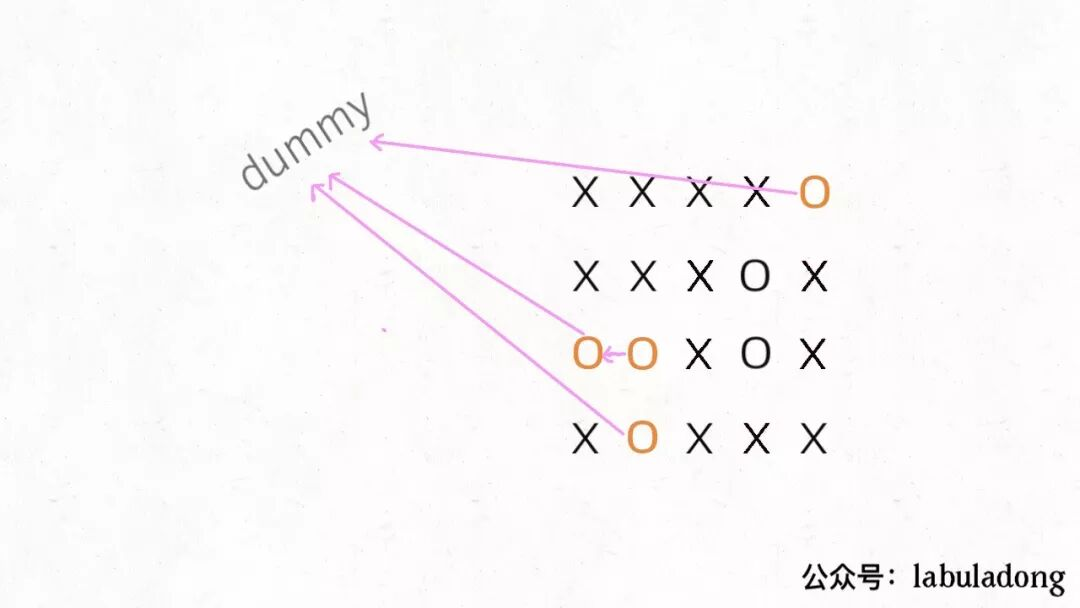## 算法详解

Union-Find 算法，也就是常说的并查集算法，主要是解决图论中「动态连通性」问题的。

1、自反性：节点`p``p`是连通的。

2、对称性：如果节点`p``q`连通，那么`q``p`也连通。

3、传递性：如果节点`p``q`连通，`q``r`连通，那么`p``r`也连通。

Union-Find 算法的关键就在于`union``connected`函数的效率

``````class UF {
// 连通分量个数
private int count;
// 存储多棵树，节点 x 的父节点是 parent[x]
private int[] parent;
// 记录树的“重量”，表示size[x] 树有几个节点
private int[] size;

public UF(int n) {
// 一开始互不连通
this.count = n;
parent = new int[n];
size = new int[n];
for (int i = 0; i < n; i++) {
// 父节点指针初始指向自己
parent[i] = i;
// 一开始每棵树只有一个节点
size[i] = 1;
}
}

/* 将 p 和 q 连接 */
public void union(int p, int q) {
int rootP = find(p);
int rootQ = find(q);
if (rootP == rootQ)
return;

// 小树接到大树下面，较平衡
if (size[rootP] > size[rootQ]) {
parent[rootQ] = rootP;
size[rootP] += size[rootQ];
} else {
parent[rootP] = rootQ;
size[rootQ] += size[rootP];
}
count--;
}

/* 判断 p 和 q 是否连通 */
public boolean connected(int p, int q) {
int rootP = find(p);
int rootQ = find(q);
return rootP == rootQ;
}

/* 返回当前的连通分量个数 */
public int count() {
return count;
}

/*路径压缩，使find能以 O(1) 的时间找到某一节点的根节点，相应的，connected和union复杂度都下降为 O(1)。*/
private int find(int x) {
while (parent[x] != x) {
// 进行路径压缩
parent[x] = parent[parent[x]];
x = parent[x];
}
return x;
}
}
``````

1、`parent`数组记录每个节点的父节点，相当于指向父节点的指针，所以`parent`数组内实际存储着一个森林（若干棵多叉树）。

2、`size`数组记录着每棵树的重量，目的是让`union`后树依然拥有平衡性，而不会退化成链表，影响操作效率。

3、`find`函数中进行路径压缩，保证任意树的高度保持在常数，使得`union``connected`API 时间复杂度为 O(1)。

## DFS 的替代方案

leetcode 130 给你一个 M×N 的二维矩阵，其中包含字符`X``O`，让你找到矩阵中完全`X`围住的`O`，并且把它们替换成`X```````void solve(char[][] board) {
if (board.length == 0) return;

int m = board.length;
int n = board.length;
// 给 dummy 留一个额外位置
UF uf = new UF(m * n + 1);
int dummy = m * n;
// 将首列和末列的 O 与 dummy 连通
for (int i = 0; i < m; i++) {
if (board[i] == 'O')
uf.union(i * n, dummy);
if (board[i][n - 1] == 'O')
uf.union(i * n + n - 1, dummy);
}
// 将首行和末行的 O 与 dummy 连通
for (int j = 0; j < n; j++) {
if (board[j] == 'O')
uf.union(j, dummy);
if (board[m - 1][j] == 'O')
uf.union(n * (m - 1) + j, dummy);
}
// 方向数组 d 是上下左右搜索的常用手法
int[][] d = new int[][]{ {1,0}, {0,1}, {0,-1}, {-1,0} };
for (int i = 1; i < m - 1; i++)
for (int j = 1; j < n - 1; j++)
if (board[i][j] == 'O')
// 将此 O 与上下左右的 O 连通
for (int k = 0; k < 4; k++) {
int x = i + d[k];
int y = j + d[k];
if (board[x][y] == 'O')
uf.union(x * n + y, i * n + j);
}
// 所有不和 dummy 连通的 O，都要被替换
for (int i = 1; i < m - 1; i++)
for (int j = 1; j < n - 1; j++)
if (!uf.connected(dummy, i * n + j))
board[i][j] = 'X';
}
``````

## 判定合法算式

``````boolean equationsPossible(String[] equations) {
// 26 个英文字母
UF uf = new UF(26);
// 先让相等的字母形成连通分量
for (String eq : equations) {
if (eq.charAt(1) == '=') {
char x = eq.charAt(0);
char y = eq.charAt(3);
uf.union(x - 'a', y - 'a');
}
}
// 检查不等关系是否打破相等关系的连通性
for (String eq : equations) {
if (eq.charAt(1) == '!') {
char x = eq.charAt(0);
char y = eq.charAt(3);
// 如果相等关系成立，就是逻辑冲突
if (uf.connected(x - 'a', y - 'a'))
return false;
}
}
return true;
}
``````